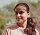How many hours overtime did a man work if he was paid \$168? - ProProfs DiscussTopicsMoreProductsMore+ Ask Question

# How many hours overtime did a man work if he was paid \$168? A man's regular pay is \$3 per hour up to 40 hours. Overtime is twice the payment for regular time.Change Image    Delete

A. 8
B. 16
C. 28
D. 48

This question is part of ECT MATHEMATICS
Asked by G.Beasley, Last updated: Jan 13, 2020

###Request 0FollowShareAnswer AnonymouslyAnswer Later Copy Link#### C. Matz

Traveler and writer by profession.

C. Matz, College student, Graduation, Orlando

The correct answer to this question is A, 8. To find this answer, one should know first multiple the pay times the number of regular hours. This would create the equation 3 x 40, which equals 120. From there, you would subtract this amount from the full amount earned. That establishes the equation 168 - 120. This gives us 48.

Then, we need to divide this amount by the hourly wage, which provides the equation with 48 / 3= 16. Being that overtime is double, we would need to use division again, this time dividing 16 /2, which gives us our answer of 8.#### S. Nicole

Content Writer, Teacher

S. Nicole, Wordsmith, PG In Journalism, New York

Here, the correct answer is option A.

Regular Pay= \$3 per hour up to 40 hours. So, total pay of the day= \$340= \$120.

He was paid\$168 for his overall work. Hence, we need to calculate overtime pay, i.e. \$168 - \$120 = \$48.

Overtime rate = 2\$3=\$6 per hour.

Therefore, the total number of overtime hours=\$48 divided by \$6 = 8.#### John Smith

John SmithAnswered Sep 14, 2016

8

At \$3 per hour up to 40 hours, regular pay = \$3 x 40 = \$120 If total pay = \$168, overtime pay = \$168 - \$120 = \$48 Overtime rate (twice regular) = 2 x \$3 = \$6 per hour ; hence number of overtime hours = \$48/\$6 = 8Search for Google imagesSelect a recommended image
Upload from your computerCancelSearch for Google imagesSelect a recommended image
Upload from your computerCancelSearch for Google imagesSelect a recommended image
Upload from your computerCancel